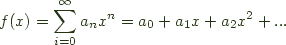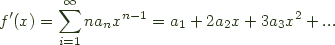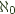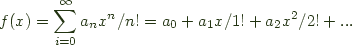Ok, I decided to take a step back from my flawed approach in the last post and play with the idea of power series of functors from a different perspective.

I dusted off my copy of Herbert Wilf's generatingfunctionology and switched goals to try to see some well known recursive functors or species as formal power series. It appears that we can pick a few things out about the generating functions of polynomial functors.

As an example:


Maybe x = 1 + x


Ok. We're done. Thank you very much. I'll be here all week. Try the veal...

For a more serious example, the formal power series for the list [x] is just a geometric series:


[x] = mu y . 1 + x y  -- the mu here is a pleasant fiction, more below
= 1 + x (1 + x (1 + x (...)))
= 1 + x + x^2 + x^3 + ...
= 1/(1-x)


Given the power series of a functor, its nth coefficient * n! tells you how many distinguishable ways its constructors can house n values. If we see that a list of n values can be permuted n! ways this has some interesting possibilities for linearizing the storage of some functors. The list case is boring, we can store a finite list of n elements by supplying the length of the array and an array of n elements, hence (among other reasons) the mu above.

Lets try decorated binary trees:


data Tree x = Leaf | Node (Tree x) x (Tree x)

Tree x = mu y. 1 + x * y * y
= 1 + x * (1 + x * (...)^2)^2
= 1 + x + 2x^2 + 5x^3 + 14x^4 + 42x^5 + ...


It turns out the coefficients of our generating function are the Catalan numbers, A000108, commonly denoted C(n), which happen to be well known for among other things, being the number of ways you can build a binary tree of n nodes.

This tells us we could store a tree by storing a number n of nodes it contains, an array of that many nodes, and an index 0 < = i < C(n) to tell you which particular tree you selected. Not that this is likely to be an incredibly time-efficient encoding, but you could then fmap over the tree by just fmapping over your array.

For a formal power series,its derivative is given by differentiating the series term by term:Consequently we can take the derivative of a list:

([]') x = 1 + 2x + 3x^2 + ... = 1/(1-x)^2 = ([] :*: []) x

and rederive the notion that a derivative/one hole context of a list can be represented by a pair of lists.

If we step slightly outside of the Haskell users' comfort zone and notion of a Functor and allow other Species, we get (as noted by apfelmus the other day) that Exp a is just a bag of elements with no predetermined order.


Exp x = 1 + x + x^2/2! + x^3/3! + ... = Bag x

Since there are n! ways to order n elements and Bag manages to forget that information, we can get a feeling for the meaning of division in this setting.

Similarly we can define:
Sinh x = x + x^3/3! + ... -- a Bag of some odd number of elements.
Cosh x = 1 + x^2/2! + ... --  a Bag of some even number of elements.


Then by construction:


Exp = Cosh :+: Sinh


The derivative of Exp is Exp, of Cosh is Sinh, of Sinh is Cosh, all as you would expect.

We can handle other species as well:


Cycle a = 1 + x^2/2 + x^3/3 + x^4/4 + ... -- cycles
Cycle_n a = x^n/n -- cycles of n elements
Bag_n a = x^n/n!  -- bags of n elements


That said, there seem to be some problems, not every functor is well behaved in this way. Lets take for instance the type of natural numbers given by the functor:


data Nat a = S (Nat a) | Z


Then the recurrence blows up, the coefficient for 0 is!

Similarly, if we parameterized a functor on another value we have to deal with the number of cases that other value can denote.


Either a x = |a| + x
(a,x) = |a| x


This is both good and bad, using the above, we can quickly establish an isomorphism between Either () and the Maybe Functor, but we blow up again for Either Integer. This gets even worse if we allow 'functors in space.' (i.e. functors that can contain functions)

On the other extreme, we might modify our tree example and remove the leaves, yielding infinite decorated cotrees.


Tree x = nu y. x * y * y
= x * (x * (...)^2)^2
= 0 + 0x + 0x^2 + 0x^3 + ...


Then a_n = 0 for all n in the natural numbers, so you can't use the coefficients of the generating function to tell you about the behavior of an infinite structure! It would appear that the generating function of a functor does not capture what happens in the greatest fixed point case, so we can only use generating functions to describe the behavior of data defined with mu, not in general codata defined by nu.

The Bags and Cycles above are nice examples, but if we wanted to rule out the non-polynomial Functors (from the Haskell perspective) in the above then we can simply limit ourselves to ordinary generating functions with natural number coefficients, that is to say generating functions of the form:To choose to admit bags, cycles and other species etc. then you need merely also permit exponential generating functions with natural coefficients, that is to say, generating functions of the form: